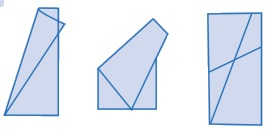Home | | Maths 6th Std | Two lines

# Two lines

Now let us get back to two lines (Fig. 4.2). Lines that go on forever on either side without meeting each other (i.e. they have a constant distance in between) are called parallel lines.

Two lines

Now let us get back to two lines (Fig. 4.2). Lines that go on forever on either side without meeting each other (i.e. they have a constant distance in between) are called parallel lines.Thus, parallel lines go forever without meeting.

What will happen if two lines are not parallel?

Then they must meet somewhere! Of course, they go their way after meeting too.Here, l1 and l2 are called intersecting lines.

Of course, we now have parallel line segments and intersecting line segments too.The position ‘O’ at which the line segmentsandmeet is called their point of intersection.

Activity

Take a piece of paper and fold in as many ways as you can, always generating parallel lines, or always generating intersecting lines.

A few examples are shown for you.Note

A line has no end points, whereas a line segment has end points. We can measure the length of a line segment.Tags : Geometry | Term 1 Chapter 4 | 6th Maths , 6th Maths : Term 1 Unit 4 : Geometry
Study Material, Lecturing Notes, Assignment, Reference, Wiki description explanation, brief detail
6th Maths : Term 1 Unit 4 : Geometry : Two lines | Geometry | Term 1 Chapter 4 | 6th Maths